# 0. Model Description

## GP model

$$t$$: age index

$$j$$: ship index

$$k[j]$$: engine index

$y_{t,j} \sim N(\mu_{t,j},\sigma)$ $\mu_{t,j} = \mu + \theta_t^{\ age}+\theta_j^{\ ship}+\theta_{k[j]}^{\ engine}+\gamma_{t,j}+\delta_{t,\ k[j]}$

$\gamma_{j} \sim \text{MultiNormal}(0,K_{l^{\gamma},{\alpha}^{\gamma}})$ $\delta_{k} \sim \text{MultiNormal}(0,K_{l^{\delta},{\alpha}^{\delta}})$ $\theta_t^{\ age} \sim N(0, {\sigma^{age}}^2)$ $\theta_j^{\ ship} \sim N(0,{\sigma^{ ship}}^2\ )$

$\theta_k^{\ engine} \sim N(0,{\sigma^{engine}}^2\ )$

## Stan Code

### data, transformed data block

data {
int<lower=1> N;
int<lower=1> N_engines;
int<lower=1> N_ships;
int<lower=1> N_ages_obs;
int<lower=1> N_ages;
int<lower=1> ship_engine_ind[N_ships];
int<lower=1,upper=99> ship_ind[N];
int<lower=1> age_ind[N];
vector[N] y;
}

transformed data {
real ages[N_ages];
int N_comp = 10;
for (t in 1:N_ages)
ages[t] = t;
}

### parameters block

parameters {
matrix[N_ages,N_engines] GP_engine_std;
matrix[N_ages,N_ships] GP_ship_std;
vector[N_ages_obs] age_std;
vector[N_ships] ship_std;
vector[N_engines] engine_std;
real<lower=0> tot_var;
simplex[N_comp] prop_var;
real mu;
real<lower=0> length_GP_engine;
real<lower=0> length_GP_ship;
real <lower = 0> length_engine_scale;
real <lower = 0> length_ship_scale;
real <lower = 0> length_engine_shape;
real <lower = 0> length_ship_shape;
}
• GP_engine_std, GP_ship_std, age_std, ship_std, engine_std follow standard normal distribution. These are tools for giving Normal prior using cholesky decomposition.

• tot_varand simplex prop_var are used to join all $$\sigma$$s in model in one vector.

• mu is $$\mu$$ above. ($$\mu_{t,j} = \mu + \theta_t^{\ age}+\theta_j^{\ ship}+\theta_{k[j]}^{\ engine}+\gamma_{t,j}+\delta_{t,\ k[j]}$$)

• length_GP_ship, length_GP_engine are length-scale of covariance matrix , i.e. $$l^{\gamma}$$, $$l^{\delta}$$.

### transformed parameters block

transformed parameters {
matrix[N_ages,N_engines] GP_engine;
matrix[N_ages,N_ships] GP_ship;

vector[N_ages_obs] age_re;
vector[N_ships] ship_re;
vector[N_engines] engine_re;
vector[N_comp] vars;

real sigma_age;
real sigma_engine;
real sigma_ship;

real sigma_error_ship;

real sigma_GP_engine;
real sigma_GP_ship;
vars = N_comp * prop_var * tot_var;
sigma_age = sqrt(vars);
sigma_engine = sqrt(vars);
sigma_ship = sqrt(vars);
sigma_GP_engine = sqrt(vars);
sigma_GP_ship = sqrt(vars);
sigma_error_ship = sqrt(vars);

engine_re = sigma_engine * engine_std;
age_re = sigma_age * age_std;
ship_re = sigma_ship * ship_std;

{
matrix[N_ages, N_ages] cov_engine;
matrix[N_ages, N_ages] cov_ship;
matrix[N_ages, N_ages] L_cov_engine;
matrix[N_ages, N_ages] L_cov_ship;

length_GP_engine);
length_GP_ship);
for (age in 1:N_ages) {
cov_engine[age, age] = cov_engine[age, age] + 1e-6;
cov_ship[age, age] = cov_ship[age, age] + 1e-6;
}

L_cov_engine = cholesky_decompose(cov_engine);
L_cov_ship = cholesky_decompose(cov_ship);
GP_engine = L_cov_engine * GP_engine_std; //f_engine
GP_ship = L_cov_ship * GP_ship_std;       //f_ship
}
}
• sigma_age, sigma_engine, sigma_ship are $$\sigma^{age}$$, $$\sigma^{engine}$$, $$\sigma^{ship}$$ above. $$\theta_t^{\ age} \sim N(0, {\sigma^{age}}^2)$$

• sigma_GP_ship, sigma_GP_engine are marginal std of covariance matrix of $$\gamma_{j}$$, $$\delta_{k}$$ i.e, $${\alpha}^{\gamma}$$, $${\alpha}^{\delta}$$.

• sigma_error_ship is $$\sigma$$ of $$y_{t,j} \sim N(\mu_{t,j},\sigma)$$.

• engine_re = sigma_engine * engine_std means $$\theta_k^{\ engine} = \sigma^{engine}\ * N(0,1)$$ so that $$\theta_k^{\ engine} \sim N(0,{\sigma^{engine}}^2\ )$$

• cov_engine, L_cov_engine are $$K_{l^{\delta},{\alpha}^{\delta}}$$ and cholesky decomposition $$L_{\delta}$$ so that $$L_{\delta}L_{\delta}^T = K_{l^{\delta},{\alpha}^{\delta}}$$

• GP_engine = L_cov_engine * GP_engine_std means $$\delta_{k} = L_{\delta}*N(0,1)$$ so that $$\delta_{k} \sim \text{MultiNormal}(0,K_{l^{\delta},{\alpha}^{\delta}})$$

### model block

model {
vector[N] obs_mu;
for (n in 1:N) {
obs_mu[n] = mu
+ age_re[age_ind[n]]                                 //fixed effects
+ engine_re[ship_engine_ind[ship_ind[n]]]
+ ship_re[ship_ind[n]]
+ GP_engine[age_ind[n],ship_engine_ind[ship_ind[n]]] //f_engine
+ GP_ship[age_ind[n],ship_ind[n]];                   //f_ship
}
y ~ normal(obs_mu, sigma_error_ship);

to_vector(GP_engine_std) ~ normal(0, 1);
to_vector(GP_ship_std) ~ normal(0, 1);
age_std ~ normal(0, 1);
ship_std ~ normal(0, 1);
engine_std ~ normal(0, 1);
mu ~ normal(.5, .5);
tot_var ~ normal(0,1);
length_engine_shape ~  normal(30, 1);
length_engine_scale ~ normal(8, 1);
length_ship_shape ~  normal(30, 1);
length_ship_scale ~ normal(3, 1);
length_GP_engine ~ weibull(length_engine_shape,length_engine_scale); // (30,8)
length_GP_ship ~ weibull(length_ship_shape,length_ship_scale); //(30,3)
}
• above paragraph means $$\mu_{t,j} = \mu + \theta_t^{\ age}+\theta_j^{\ ship}+\theta_{k[j]}^{\ engine}+\gamma_{t,j}+\delta_{t,\ k[j]}$$

• y ~ normal(obs_mu, sigma_error_ship) means $$y_{t,j} \sim N(\mu_{t,j},\sigma)$$

• GP_engine_std, GP_engine_std, age_std, ship_std, engine_std follow standard normal because they are used to give normal dist with cholesky.

• priors for $$\mu$$, $$\frac{\text{sum of all sigma s}}{6}$$, $$l^{\gamma}$$, $$l^{\delta}$$ are given.

util <- new.env()
source('stan_utility.R', local=util)
source('gp_utility.R', local=util)
library(rstan); library(cmdstanr); library(parallel); library("tidyverse"); library(dplyr)
## Warning: package 'rstan' was built under R version 3.6.3
## Warning: package 'StanHeaders' was built under R version 3.6.3
## Warning: package 'ggplot2' was built under R version 3.6.3
## Warning: package 'tidyverse' was built under R version 3.6.3
## Warning: package 'tibble' was built under R version 3.6.3
## Warning: package 'tidyr' was built under R version 3.6.3
## Warning: package 'readr' was built under R version 3.6.3
## Warning: package 'purrr' was built under R version 3.6.3
## Warning: package 'dplyr' was built under R version 3.6.3
## Warning: package 'stringr' was built under R version 3.6.3
## Warning: package 'forcats' was built under R version 3.6.3
setwd(getwd())
set.seed(1954)
.libPaths("~/Rlib")
options(mc.cores = parallel::detectCores())
util <- new.env()
source('stan_utility.R', local=util)
source('gp_utility.R', local=util)

c_light <- c("#DCBCBC")
c_light_highlight <- c("#C79999")
c_mid <- c("#B97C7C")
c_mid_highlight <- c("#A25050")
c_dark <- c("#8F2727")
c_dark_highlight <- c("#7C0000")

c_light_trans <- c("#DCBCBC80")
c_light_highlight_trans <- c("#C7999980")
c_mid_trans <- c("#B97C7C80")
c_mid_highlight_trans <- c("#A2505080")
c_dark_trans <- c("#8F272780")
c_dark_highlight_trans <- c("#7C000080")

c_light_teal="#6B8E8E"
c_mid_teal="#487575"
c_dark_teal="#1D4F4F"

c_green_trans <- c("#00FF0080")
c_superfine <- c("#8F272705")

println <- function(msg) cat(msg); cat("\n") 
printf <- function(pattern, ...) println(sprintf(pattern, ...))

scriptDir <- getwd()
modelDir <- file.path(scriptDir, "models")
gp_fit <- function(modelName, data){
chains <- 4
parallel_chains <- min(chains, detectCores())
scriptDir <- getwd()
delivDir <- file.path(scriptDir, "deliv", modelName)
prefit <- file.path(delivDir, paste0(modelName, ".rda"))
stanfile <- file.path(modelDir, modelName, paste0(modelName, ".stan"))

if (file.exists(prefit)){
}else{
mod <- cmdstan_model(stanfile, quiet = FALSE)
fit <- mod$sample(data, chains = chains, iter_warmup = 500, iter_sampling = 500, parallel_chains = parallel_chains, save_warmup = FALSE) dir.create(delivDir) fit$save_object(file = prefit)
}
fit
}

div_detect <- function(stanfit){
partition <- util$partition_div(stanfit) div_samples <- partition[] nondiv_samples <- partition[] par(mfrow=c(1, 3)) plot(nondiv_samples$length_GP_engine, nondiv_samples$sigma_GP_engine, log="xy", col=c_dark_trans, pch=16, cex=0.8, xlab="length_GP_engine", ylab="sigma_GP_engine") points(div_samples$length_GP_engine, div_samples$sigma_GP_engine, col=c_green_trans, pch=16, cex=0.8) plot(nondiv_samples$length_GP_engine, nondiv_samples$sigma_error_ship, col=c_dark_trans, pch=16, cex=0.8, xlab="length_GP_engine", ylab="sigma_error_ship") points(div_samples$length_GP_engine, div_samples$sigma_error_ship, col=c_green_trans, pch=16, cex=0.8) plot(nondiv_samples$length_engine_scale, nondiv_samples$length_GP_engine_s, col=c_dark_trans, pch=16, cex=0.8, xlab="length_engine_scale", ylab="length_GP_engine_s") points(div_samples$length_engine_scale, div_samples$length_GP_engine_s, col=c_green_trans, pch=16, cex=0.8) } data preparation N_engines <- 5 N_ships <- 99 N_ages <- 31 N_ages_obs <- 31 ship_engine_ind <- read.csv("data/engine.csv")$engine
ship_ind <- read.csv("data/ship_index.csv")$X0 age_ind <- read.csv("data/x_age.csv", header = FALSE)[-1,1] y <- read.csv("data/y_count_pwr.csv", header = FALSE)[,1] data <- list(N = length(y), N_engines=N_engines,N_ships = N_ships, N_ages= N_ages, N_ages_obs = N_ages_obs, ship_engine_ind =ship_engine_ind, ship_ind = ship_ind, age_ind = age_ind, y=y) mseNplot <- function(x, y){ yhat<- x %>% dplyr::filter(str_detect(variable, "y_new_pred")) %>% pull(mean) yhat<- (matrix(yhat, nrow = 31, ncol = 99)) y_hat <- rep(NA, length(y)) for (i in 1:length(y)){ y_hat[i] <- yhat[age_ind[i],ship_ind[i]] } par(mfrow=c(1,1)) plot(1, type="n",xlim=c(0,31),ylim=c(-3,4),xlab="age",ylab="scaled failure count") for (n in 1:653){ points(age_ind[n],y[n],col="black",pch=16) points(age_ind[n],y_hat[n],col="red") } mean((y-y_hat)^2) } We have started from this reference code and modified based on the following steps. 1.domain knowledge, 2.prior pushforwd check, 3.prior predictive check. The last two differ in that the former’s stage is parameter space and the latter is observation, i.e. y, space. # 1. domain knowledge First modifications were to simplify the model. 1. remove prop prior 2. unify trend from long and short trend 3. parameterize the length_engine_ship prior parameters $$\alpha$$ and $$\rho$$ parameters could tuned with algebraic solvers match one’s domain knowledge (such as INLA example from Talts, 2018 or Mike Bentancourt’s casestudy) but as we did not have domain knowledge on length_engine_ship we decided to give prior to its scale and location parameter. length_GP_engine ~ weibull(length_engine_shape,length_engine_scale); length_GP_ship ~ weibull(length_ship_shape,length_ship_scale); gp_hp.stan is our baseline code. # 2. pre-predictive check Prametrizing prevented the need for our domain knowledge but instead, we had to set reasonable prior for length_GP_engine and length_GP_ship. First attemps were just to experiment with the given parmameters in the orginal model; which ended up with divergences. > length_engine_shape ~ normal(30, 1); length_engine_scale ~ normal(8, 1); length_ship_shape ~ normal(30, 1); length_ship_scale ~ normal(3, 1); modelName <- "gp_hp" sf_hp <- gp_fit(modelName, data) rsf_hp <- read_stan_csv(sf_hp$output_files())
util$check_all_diagnostics(rsf_hp) ##  "n_eff / iter looks reasonable for all parameters" ##  "Rhat looks reasonable for all parameters" ##  "4 of 2000 iterations ended with a divergence (0.2%)" ##  " Try running with larger adapt_delta to remove the divergences" ##  "0 of 2000 iterations saturated the maximum tree depth of 10 (0%)" ##  "E-FMI indicated no pathological behavior" partition <- util$partition_div(rsf_hp)
div_samples <- partition[]
nondiv_samples <- partition[]

par(mfrow=c(1, 3))
plot(nondiv_samples$length_GP_engine, nondiv_samples$sigma_GP_engine, log="xy",
col=c_dark_trans, pch=16, cex=0.8, xlab="length_GP_engine", ylab="sigma_GP_engine")
points(div_samples$length_GP_engine, div_samples$sigma_GP_engine,
col=c_green_trans, pch=16, cex=0.8)

plot(nondiv_samples$length_GP_engine, nondiv_samples$sigma_error_ship,
col=c_dark_trans, pch=16, cex=0.8, xlab="length_GP_engine", ylab="sigma_error_ship")
points(div_samples$length_GP_engine, div_samples$sigma_error_ship,
col=c_green_trans, pch=16, cex=0.8)

plot(nondiv_samples$length_GP_engine, nondiv_samples$length_engine_scale,
col=c_dark_trans, pch=16, cex=0.8, xlab="length_GP_engine", ylab="length_engine_scale")
points(div_samples$length_GP_engine, div_samples$length_engine_scale,
col=c_green_trans, pch=16, cex=0.8)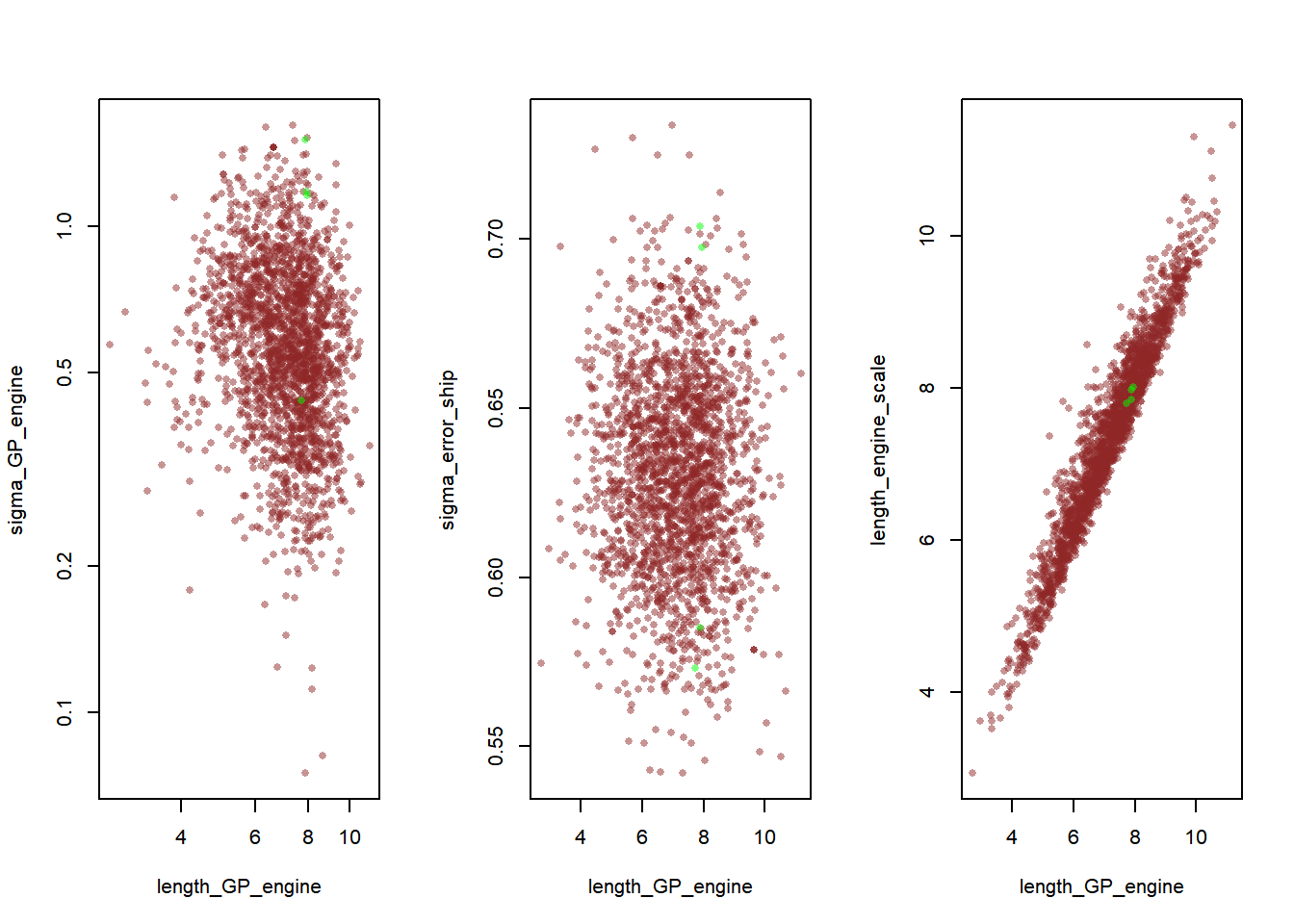We observed two problems from the results.

## 2.1. correlated and uneven density

When plotted, length_GP_engine and length_engine_scale showed ununiform samples which can be problematic while sampling discourse discussion, so we decided to reparameterize length_GP_engine.

partition <- util$partition_div(rsf_hp) div_samples <- partition[] nondiv_samples <- partition[] par(mfrow=c(1, 2)) plot(nondiv_samples$length_engine_scale, nondiv_samples$length_GP_engine, log="xy", col=c_dark_trans, pch=16, cex=0.8, xlab="length_engine_scale", ylab="length_GP_engine") points(div_samples$length_engine_scale, div_samples$length_GP_engine, col=c_green_trans, pch=16, cex=0.8)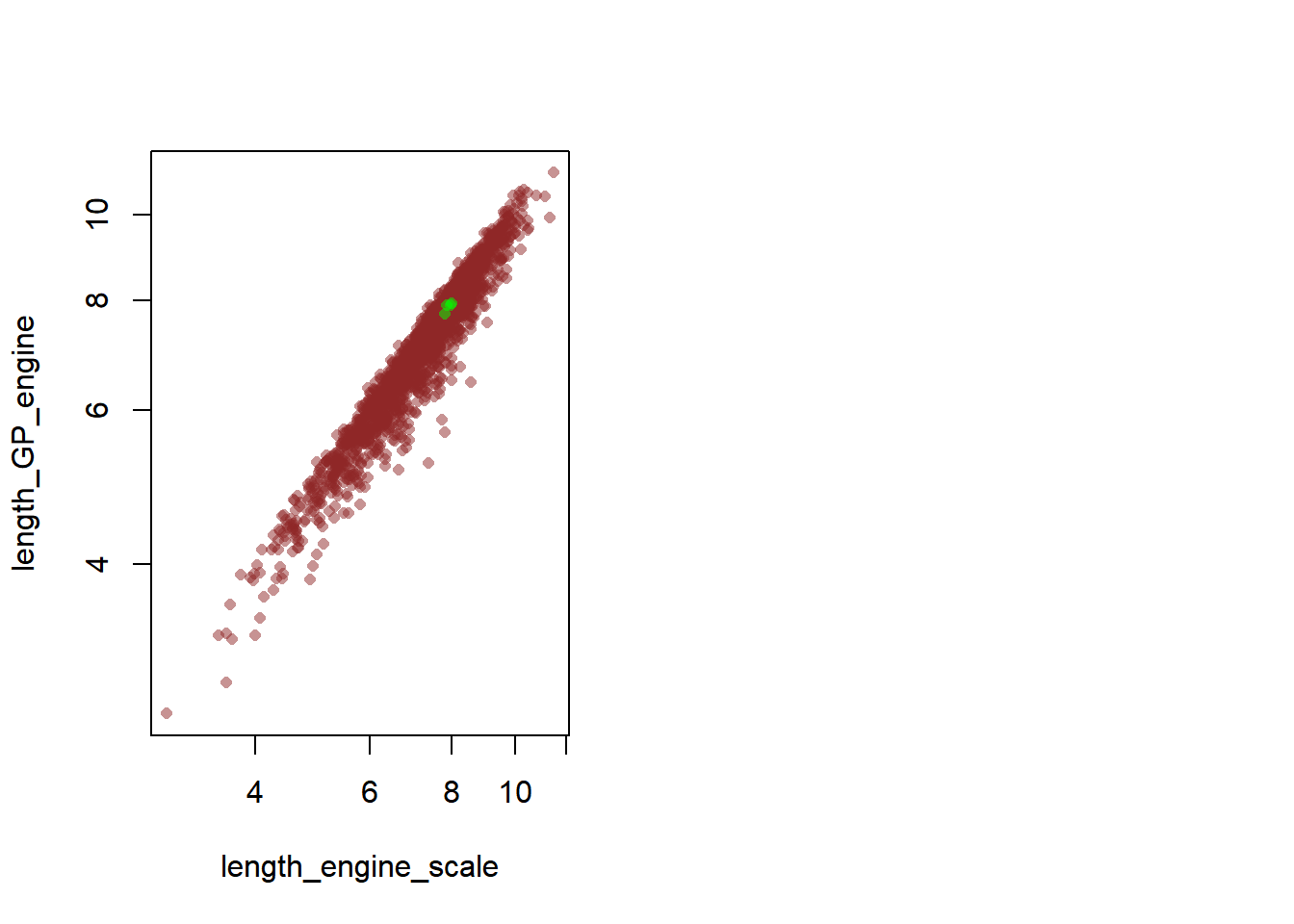Since mode and mean largely depends on scale parameter in Weibull distribution, we reparametrized length_GP_engine so that scale parameter is fixed to 1. $\rho_{engine} \sim \text{Weibull}(\alpha_{\rho},\sigma_{\rho})$ is equivalent to $\rho_{engine}^s \sim \text{Weibull}(\alpha_{\rho},1)$ $\rho_{engine} = \rho_{engine}^s * \sigma_{\rho}$ This is because $$Y \sim \text{Weibull}(\alpha,\sigma)$$ $$\Rightarrow$$ $$kY \sim \text{Weibull}(\alpha,k\sigma)$$ from length_GP_engine ~ weibull(length_engine_shape,length_engine_scale) length_GP_ship ~ weibull(length_engine_ship,length_engine_ship) to real length_GP_engine = length_engine_scale * length_GP_engine_s; real length_GP_ship = length_ship_scale * length_GP_ship_s; length_GP_engine_s ~ weibull(length_engine_shape,1); length_GP_ship_s ~ weibull(length_ship_shape,1);  modelName <- "gp_reparam" sf_reparam <- gp_fit(modelName, data) rsf_reparam <- read_stan_csv(sf_reparam$output_files())
util$check_all_diagnostics(rsf_reparam) ##  "n_eff / iter looks reasonable for all parameters" ##  "Rhat looks reasonable for all parameters" ##  "0 of 2000 iterations ended with a divergence (0%)" ##  "0 of 2000 iterations saturated the maximum tree depth of 10 (0%)" ##  "E-FMI indicated no pathological behavior" div_detect(rsf_reparam)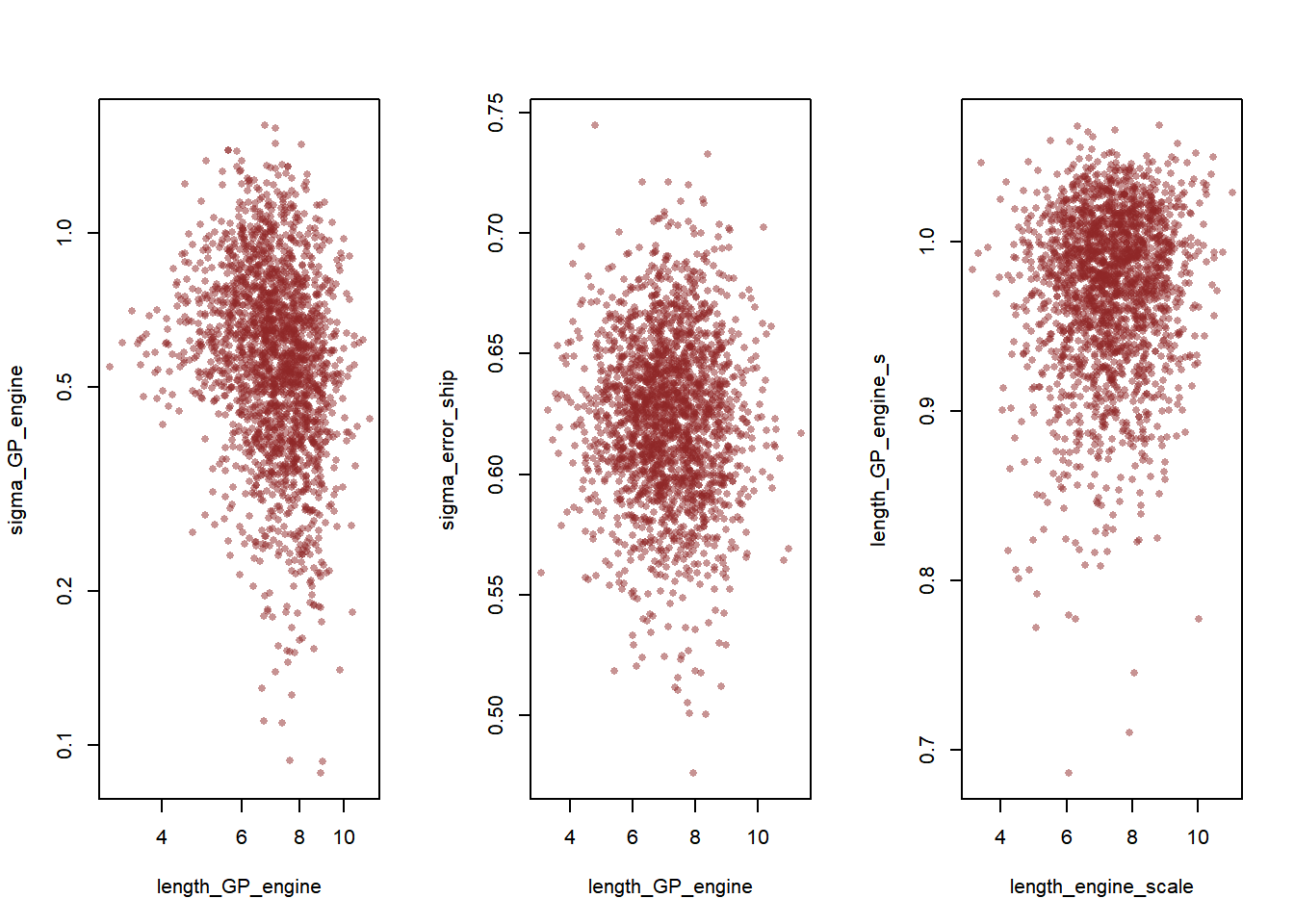partition <- util$partition_div(rsf_reparam)
div_samples <- partition[]
nondiv_samples <- partition[]

par(mfrow=c(1, 3))
plot(nondiv_samples$length_engine_scale, nondiv_samples$length_GP_engine_s, log="xy",
col=c_dark_trans, pch=16, cex=0.8, xlab="length_engine_scale", ylab="length_GP_engine_s")
points(div_samples$length_engine_scale, div_samples$length_GP_engine_s,
col=c_green_trans, pch=16, cex=0.8)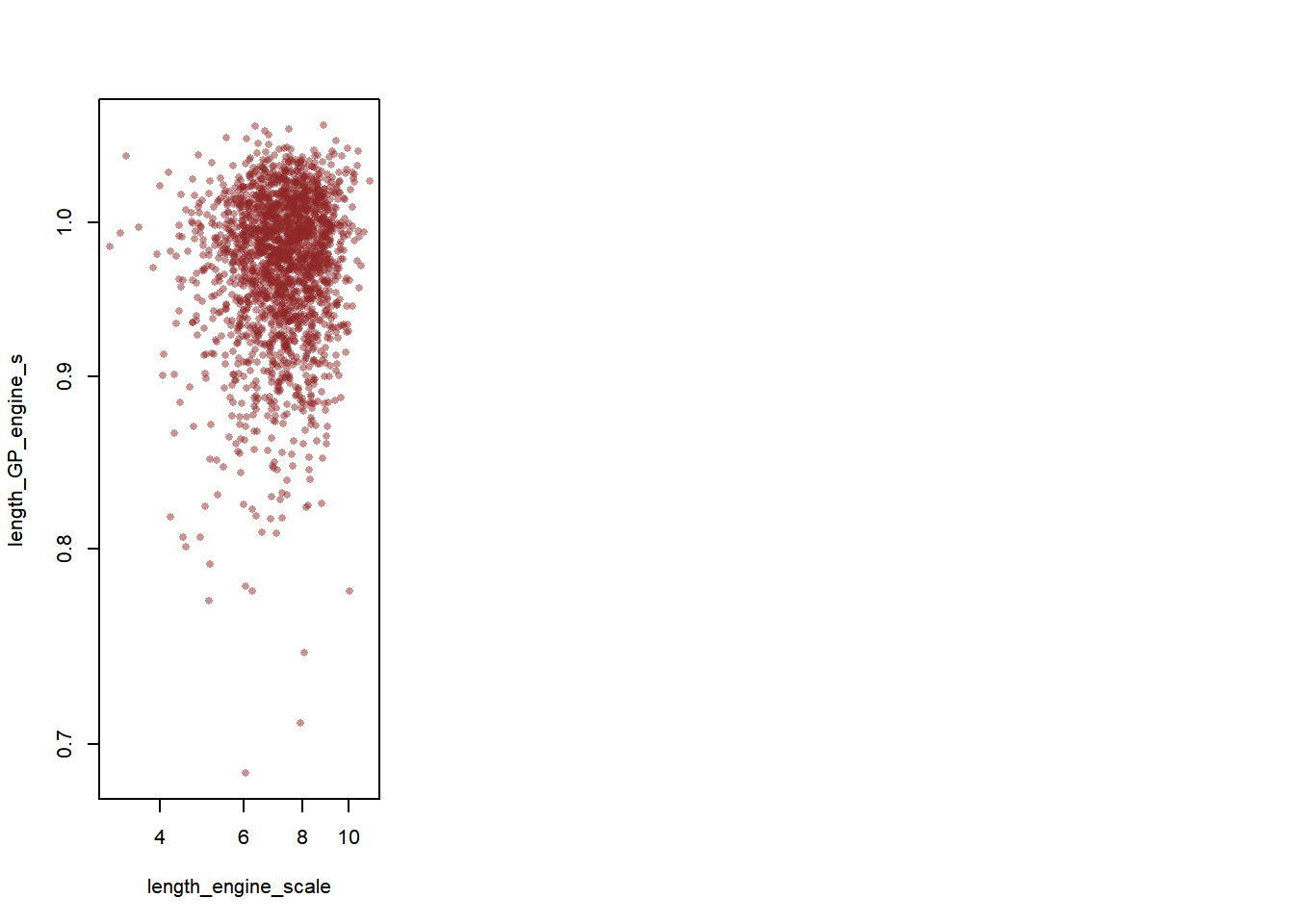The reparameterization has led to more even plot.

## 2.2. identification problems

As can be seen from the result, the value does not change much from the given prior values, which could indicate identification issues (refer to Bentancourt’s identity crisis). This might be due to the fact that data are scaled for security issues and have relatively weak impact on the prior update@. Therefore, to investigate the parameters’ true range, weak priors are given. We chose $$lognormal(0,3)$$.

length_engine_scale ~ lognormal(0, 3);
length_ship_scale ~ lognormal(0, 3);
length_engine_shape ~  lognormal(0, 3);
length_ship_shape ~  lognormal(0, 3);
modelName <- "gp_hp_lognorm"
data$hp_scale <- 3 sf_hp_ln <- gp_fit(modelName, data) rsf_hp_ln <- read_stan_csv(sf_hp_ln$output_files())
util$check_all_diagnostics(rsf_hp_ln) ##  "n_eff / iter looks reasonable for all parameters" ##  "Rhat for parameter length_GP_ship is 1.13027917437218!" ##  " Rhat above 1.1 indicates that the chains very likely have not mixed" ##  "271 of 2000 iterations ended with a divergence (13.55%)" ##  " Try running with larger adapt_delta to remove the divergences" ##  "349 of 2000 iterations saturated the maximum tree depth of 10 (17.45%)" ##  " Run again with max_treedepth set to a larger value to avoid saturation" ##  "E-FMI indicated no pathological behavior" div_detect(rsf_hp_ln)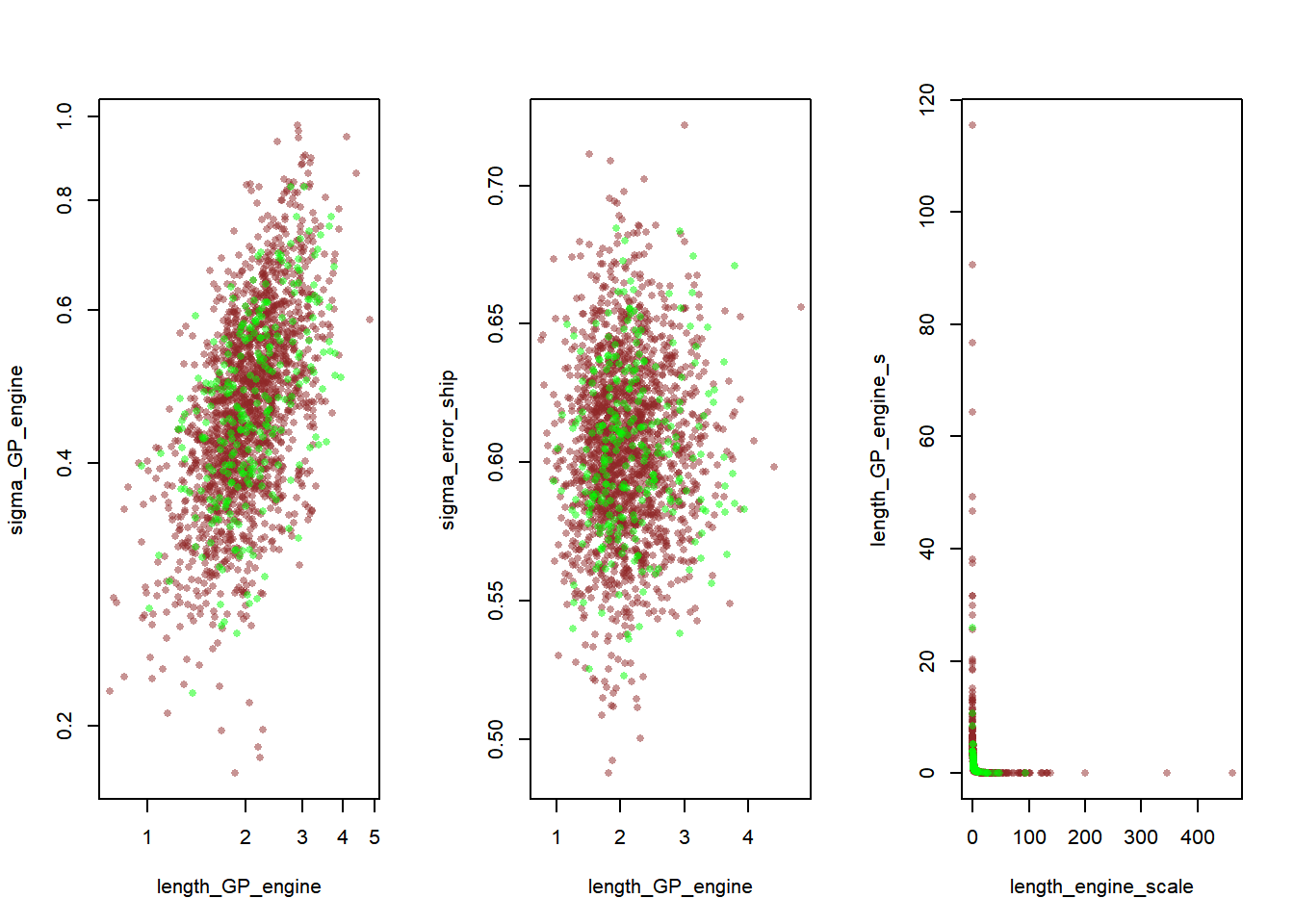emp_le_shape <-sf_hp_ln$summary("length_engine_shape")%>% pull(mean)
emp_le_scale <-sf_hp_ln$summary("length_engine_scale")%>% pull(mean) emp_ls_shape <-sf_hp_ln$summary("length_ship_shape")%>% pull(mean)
emp_ls_scale <-sf_hp_ln$summary("length_ship_scale")%>% pull(mean) print(c(emp_le_shape, emp_le_scale, emp_ls_shape,emp_ls_scale)) ##  18.725967 5.773572 11.603859 8.269962 Restricting the range of length_engine_* and length_ship_* is needed to address divergence problems. We decided to plug in the empirical values. modelName <- "gp_hp_norm" data$hp_scale <- 1
data$emp_le_shape <- emp_le_shape data$emp_le_scale <- emp_le_scale
data$emp_ls_shape <- emp_ls_shape data$emp_ls_scale <- emp_ls_scale
sf_hp_n <- gp_fit(modelName, data)
rsf_hp_n <- read_stan_csv(sf_hp_n$output_files()) util$check_all_diagnostics(rsf_hp_n)
##  "n_eff / iter looks reasonable for all parameters"
##  "Rhat looks reasonable for all parameters"
##  "0 of 2000 iterations ended with a divergence (0%)"
##  "0 of 2000 iterations saturated the maximum tree depth of 10 (0%)"
##  "E-FMI indicated no pathological behavior"
div_detect(rsf_hp_n)